# Scatter Diagram

## Scatter Diagram – Definition

A scatter diagram is used to examine the relationship between both the axes (X and Y) with one variable. In the graph, if the variables are correlated, then the point drops along a curve or line. A scatter diagram or scatter plot gives an idea of the nature of relationship.

In a scatter correlation diagram, if all the points stretch in one line, then the correlation is perfect and is in unity. However, if the scatter points are widely scattered throughout the line, then the correlation is said to be low. If the scatter points rest near a line or on a line, then the correlation is said to be linear.

 Q.1. From the following data, draw a scatter diagram and state the type of correlation between the variables X and Y. X 1 2 3 4 5 Y 5 10 15 20 25
 Q.2. From the following data, draw a scatter diagram and state the type of correlation between the variables X and Y. X 1 2 3 4 5 Y 5 10 15 20 25
 Q.3. From the following data, draw a scatter diagram and state the type of correlation between the variables X and Y. X 1 2 3 4 5 Y 5 10 15 20 25

Scatter Diagram

Perfect Positive Correlation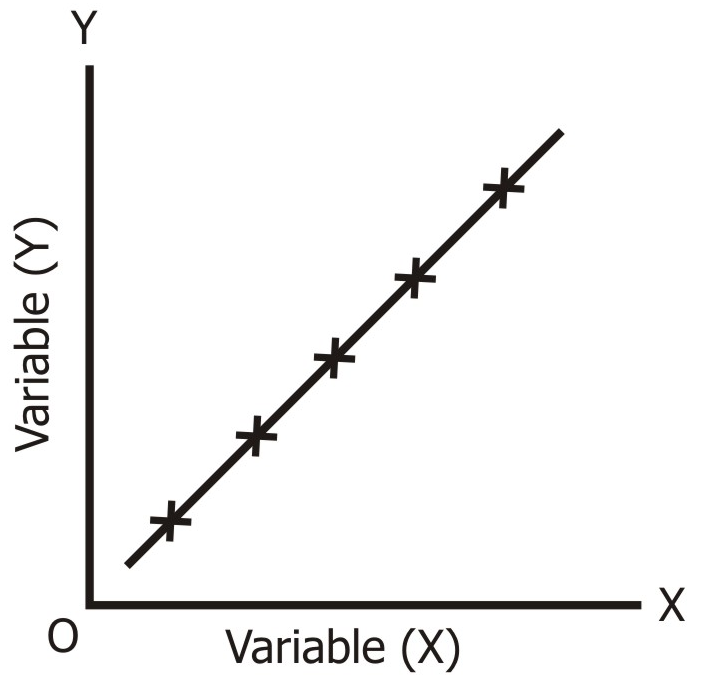Perfect Negative Correlation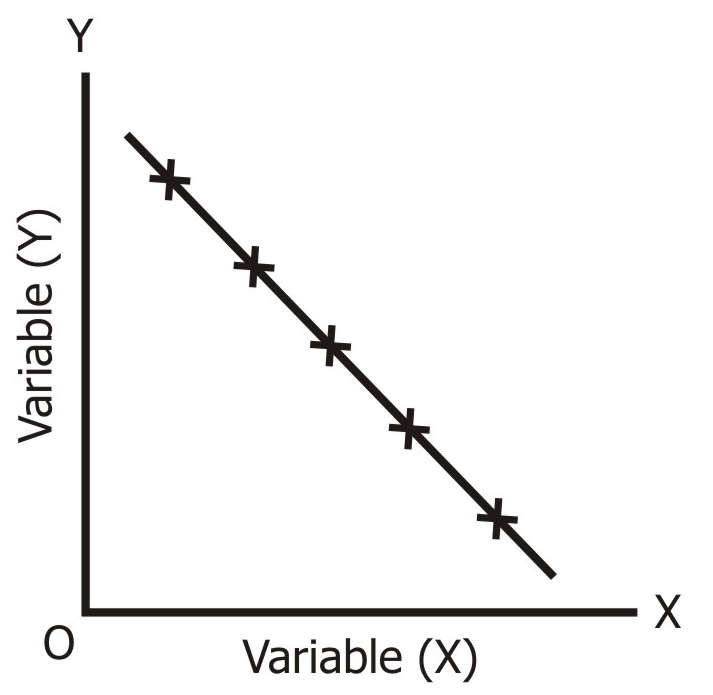High Degree of Positive Correlation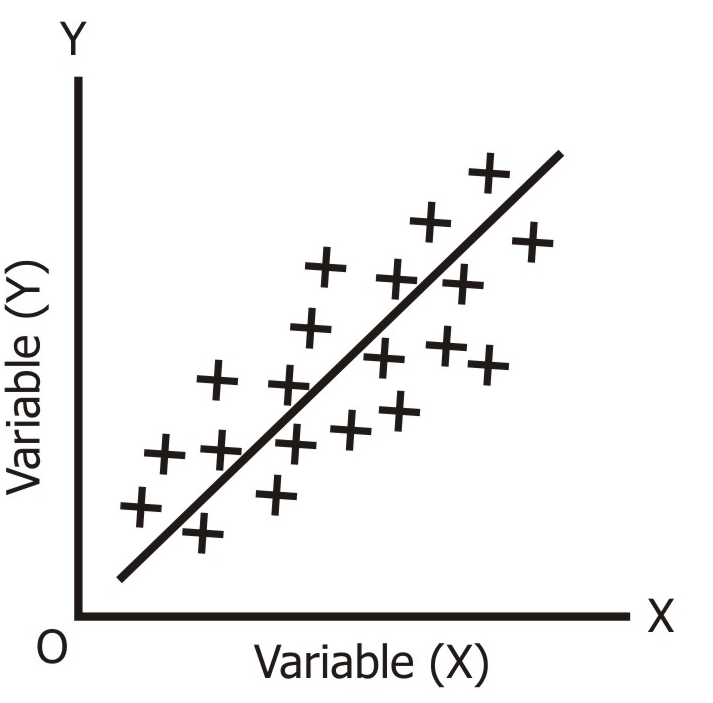High Degree of Negative Correlation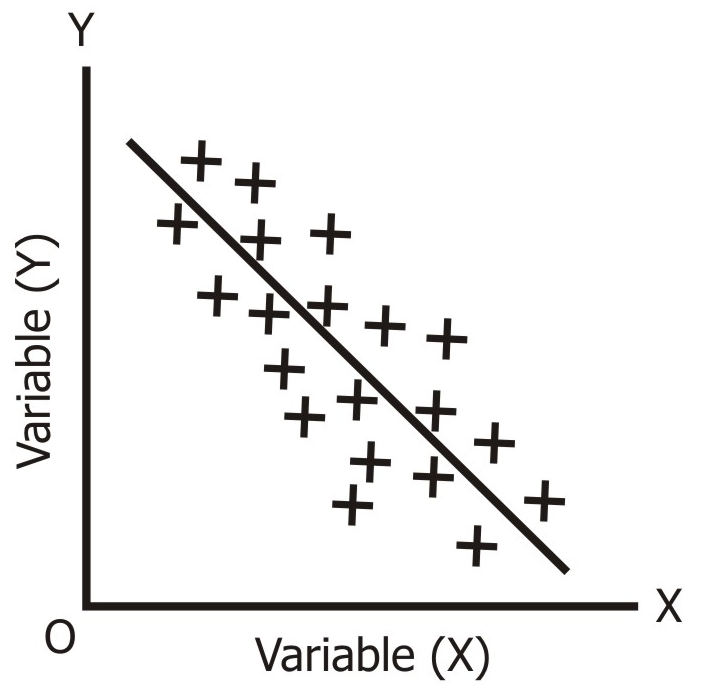Low Degree of Positive Correlation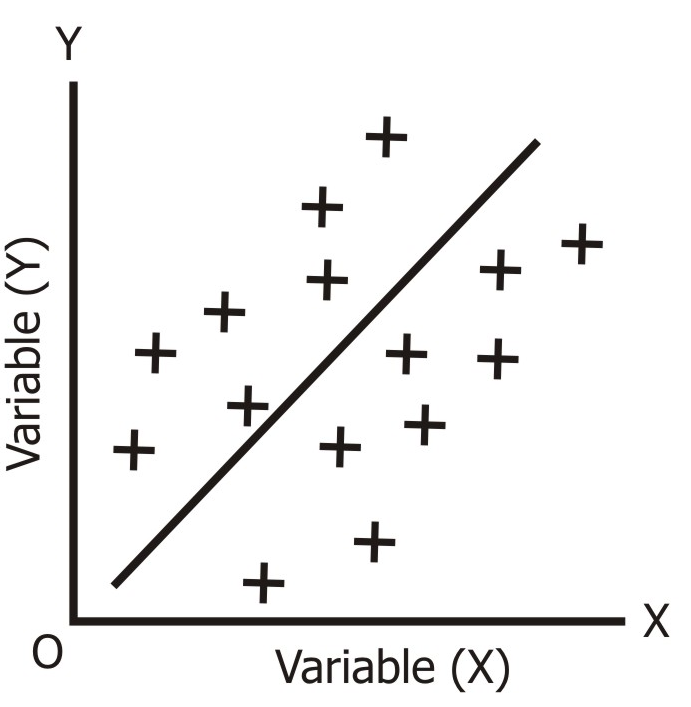Low Degree of Negative Correlation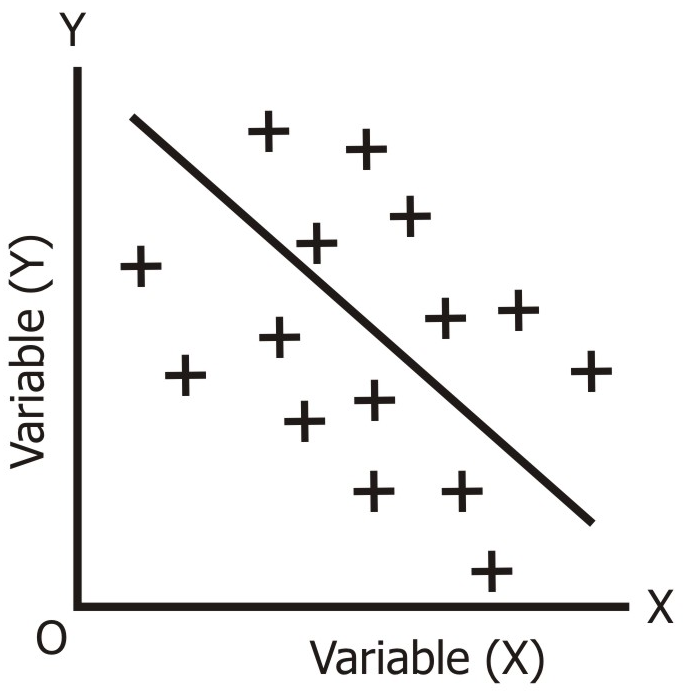No Correlation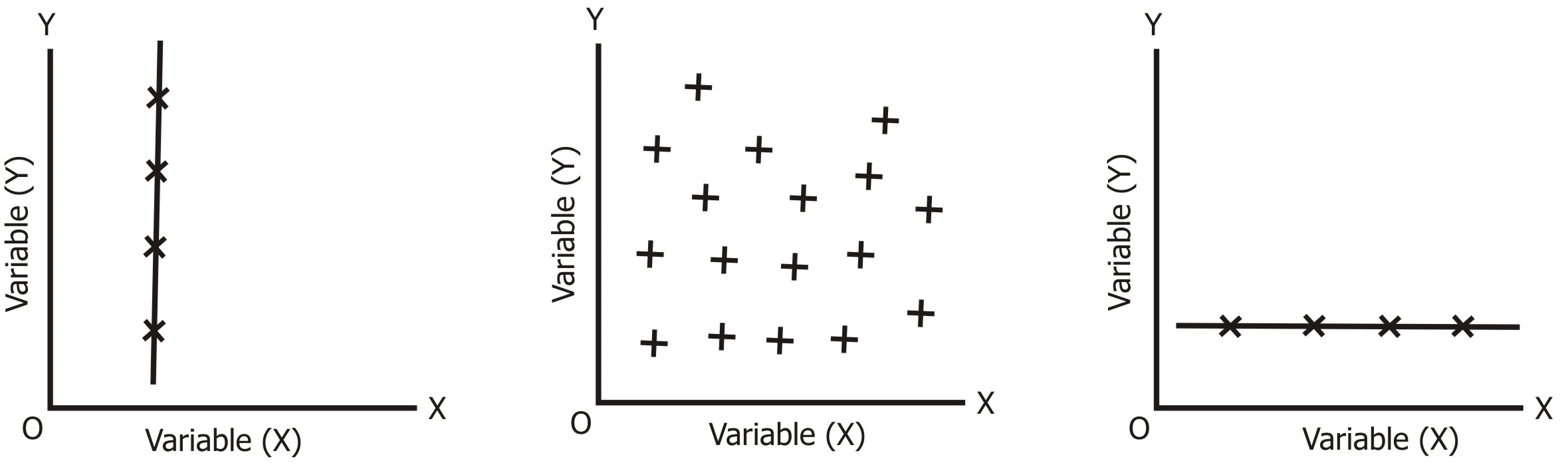This was all about the concept of scatter diagram, for more such concepts, stay tuned to our website.